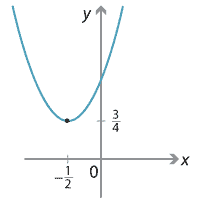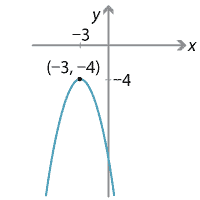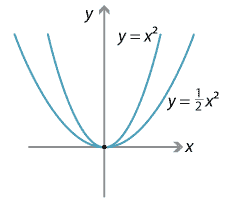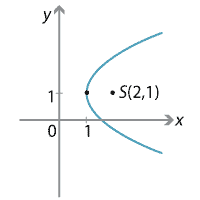## Answers to exercises

##### Exercise 1

abDetailed description of diagram

##### Exercise 2

The arms of the parabola $$y=x^2$$ are steeper.Detailed description of diagram

##### Exercise 3
$$y=2(x-1)^2+3$$
##### Exercise 4

The discriminant is $$36k^2-16(4k+1)$$ and we set this equal to zero in order to obtain one real solution to the given equation. This gives $$9k^2-16k-4=0$$, which has solutions $$k=2$$ or $$k= -\dfrac{2}{9}$$.

##### Exercise 5

The discriminant is $$\Delta = 36k^2$$. One real solution occurs when $$\Delta=0$$, which is when $$k=0$$. The quadratic is never negative definite, since $$\Delta \geq 0$$ for all values of $$k$$.

##### Exercise 6

Divide the wire into $$4x$$cm for the circle and $$(20-4x)$$cm for the square. (The 4 is to make the algebra easier.) The total area is then

$S=\dfrac{4x^2}{\pi} + (5-x)^2.$

Since the leading coefficient is positive, the parabola has a minimum. We set $$\dfrac{dS}{dx}=0$$ to find the stationary point, which occurs when $$x= \dfrac{5\pi}{4+\pi}$$. Hence, we use $$\dfrac{20\pi}{4+\pi}$$cm for the circle and $$\dfrac{80}{4+\pi}$$cm for the square.

##### Exercise 7

$$x^2-4x-41$$

##### Exercise 8
• a $$\dfrac{4}{7}$$
• b $$-\dfrac{12}{49}$$
##### Exercise 9

We have

\begin{align*} (\alpha-\beta)^2 &= (\alpha+\beta)^2 - 4\alpha\beta\\ &= \Big({-}\dfrac{b}{a}\Big)^2 - \dfrac{4c}{a} = \dfrac{b^2-4ac}{a^2}. \end{align*}

Note that, if $$a=1$$, then $$(\alpha-\beta)^2$$ is the discriminant of the quadratic. Similarly, we can define the discriminant of a monic cubic with roots $$\alpha, \beta, \gamma$$ to be $$(\alpha-\beta)^2(\alpha-\gamma)^2(\beta-\gamma)^2$$, and so on for higher degree equations.

##### Exercise 10

The arithmetic mean is $$\dfrac{17}{6}$$ and the geometric mean is 2. (Note that the actual roots are $$\dfrac{17\pm \sqrt{145}}{6}$$ !)

##### Exercise 11

Successively substitute $$x=1$$, $$x=2$$, $$x=3$$ and solve the resulting equations for $$a,b,c$$ to obtain $$x^2=3(x-1)^2-3(x-2)^2+(x-3)^2$$.

##### Exercise 12

Substituting the line into the circle we obtain $$x^2-4x+4=0$$. The discriminant of this quadratic is 0 and so the line is tangent to the circle at $$(2,1)$$.

##### Exercise 13

The parabola can be written as $$(y - 1)^2 = 4(x - 1)$$. So the vertex is $$(1,1)$$, the focal length is $$a=1$$, the focus is $$(2,1)$$, and the $$y$$-axis is the directrix.Detailed description of diagram

##### Exercise 14

The line $$3x+2y=1$$.

##### Exercise 15

If $$PQ$$ is a focal chord, then $$pq=-1$$. Hence we can write the coordinates of $$Q$$ as $$\big({-}\dfrac{2a}{p}, \dfrac{a}{p^2}\big)$$. Thus

\begin{align*} PQ^2 &= \Big(2ap + \dfrac{2a}{p}\Big)^2 + \Big(ap^2 - \dfrac{a}{p^2}\Big)^2\\ &= 4a^2p^2 + 8a^2 + \dfrac{4a^2}{p^2} + a^2p^4 - 2a^2 + \dfrac{a^2}{p^4}\\ &= a^2\Big(p^4 + 4p^2 + 6 + \dfrac{4}{p^2} + \dfrac{1}{p^4}\Big)\\ &= a^2\Big(p + \dfrac{1}{p}\Big)^4, \end{align*}

where we recall the numbers $$1,4,6,4,1$$ from Pascal's triangle and the binomial theorem. So $$PQ = a\Big(p+\dfrac{1}{p}\Big)^2$$.

##### Exercise 16

The equations of the normals at $$P$$ and $$Q$$ are respectively

$x+py = 2ap+ap^3 \qquad \text{and} \qquad x+qy = 2aq+aq^3.$

Subtracting we have

$y(p-q) = a(p^3-q^3)+2a(p-q) = a(p-q)(p^2+q^2+pq+2)$

so $$y=a(p^2+q^2+pq+2)$$. Substituting back we have $$x=-apq(p+q)$$ after simplifying. Hence $$R$$ is the point $$\big({-}apq(p+q), a(p^2+q^2+pq+2)\big)$$.

Now, if $$PQ$$ is a focal chord, then $$pq=-1$$. Thus the $$x$$- and $$y$$-coordinates of $$R$$ become $$x=a(p+q)$$ and $$y = a(p^2+q^2+1) = a\big((p+q)^2+3\big)$$, since $$pq=-1$$. We can then eliminate $$p$$ and $$q$$ to obtain

$y=a\Bigg(\Big(\dfrac{x}{a}\Big)^2 + 3\Bigg) = \dfrac{x^2}{a} + 3a,$

which is a parabola.

##### Exercise 17

Squaring

$\sqrt{(x-c)^2+y^2}= 2a - \sqrt{(x+c)^2+y^2},$

we have

$a\sqrt{(x+c)^2+y^2} = a^2+xc.$

Squaring again and rearranging, we arrive at

$x^2(a^2-c^2) + a^2y^2= a^2(a^2-c^2).$

Dividing by $$a^2(a^2-c^2)$$ gives the desired equation.